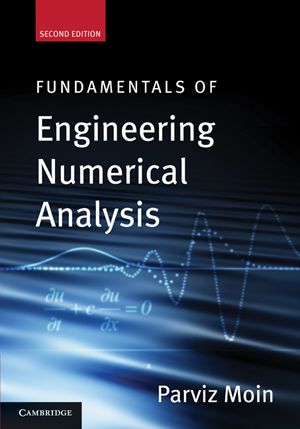# Fundamentals of Engineering Numerical Analysis

### Parviz Moin

2nd Edition | August 2010 | 256 pages 64 line diagrams | Cambridge University Press (Paperback | Hardback)

Since the original publication of this book, available computer power has increased greatly. Today, scientific computing is playing an ever more prominent role as a tool in scientific discovery and engineering analysis. In this second edition, the key addition is an introduction to the finite element method. This is a widely used technique for solving partial differential equations (PDEs) in complex domains. This text introduces numerical methods and shows how to develop, analyze, and use them. Complete MATLAB programs for all the worked examples are now available at www.cambridge.org/Moin, and more than 30 exercises have been added. This thorough and practical book is intended as a first course in numerical analysis, primarily for new graduate students in engineering and physical science. Along with mastering the fundamentals of numerical methods, students will learn to write their own computer programs using standard numerical methods.

### Contents

Preface
1. Interpolation
2. Numerical differentiation - finite differences
3. Numerical integration
4. Numerical solution of ordinary differential equations
5. Numerical solution of partial differential equations
6. Discrete transform methods
Appendix. A review of linear algebra